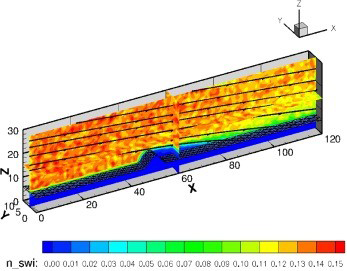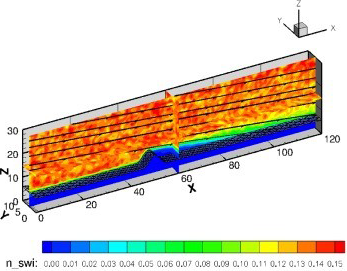# Mathematics

### Computationally Intensive Modelling in Mathematics and Statistics(a) Non-homogeneous flux jump condition(b) Homogeneous flux jump condition

The standard method to simulate collisionless plasma is known as the Particle-In-Cell (PIC). We have developed a parallel finite element PIC method for different important problems of charging in space, such as the electrostatic levitation of lunar dust and ion thrusters. To address a group of large scale plasma simulation problems, this research combines mathematical modeling, efficient parallelization, benchmark studies, mathematical analysis, and engineering applications into a hybrid system of research which will fully take advantage of the intensive computation as well as the inherent interaction among the mathematics and engineering problems.

### Principal Investigator

##### Xiaoming He

Associate Professor

Research Interests

Interface problems, computational fluid dynamics, computational electromagnetics, stochastic PDEs, boundary integral equations, feedback control

Finite element methods, domain decomposition methods, extrapolations

Computational plasma physics (Particle-In-Cell method)